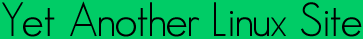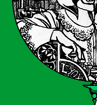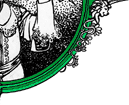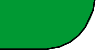Quick ?s
Cheat Sheets
Man Pages
The Lynx
Software
 ```FPCLASSIFY(3) Linux Programmers Manual FPCLASSIFY(3) NAME fpclassify, isfinite, isnormal, isnan, isinf - floating-point classifi cation macros SYNOPSIS #include int fpclassify(x); int isfinite(x); int isnormal(x); int isnan(x); int isinf(x); Link with -lm. Feature Test Macro Requirements for glibc (see feature_test_macros(7)): fpclassify(), isfinite(), isnormal(): _XOPEN_SOURCE >= 600 || _ISOC99_SOURCE; or cc -std=c99 isnan(): _BSD_SOURCE || _SVID_SOURCE || _XOPEN_SOURCE || _ISOC99_SOURCE; or cc -std=c99 isinf(): _BSD_SOURCE || _SVID_SOURCE || _XOPEN_SOURCE >= 600 || _ISOC99_SOURCE; or cc -std=c99 DESCRIPTION Floating point numbers can have special values, such as infinite or NaN. With the macro fpclassify(x) you can find out what type x is. The macro takes any floating-point expression as argument. The result is one of the following values: FP_NAN x is "Not a Number". FP_INFINITE x is either plus or minus infinity. FP_ZERO x is zero. FP_SUBNORMAL x is too small to be represented in normalized format. FP_NORMAL if nothing of the above is correct then it must be a normal floating-point number. The other macros provide a short answer to some standard questions. isfinite(x) returns a non-zero value if (fpclassify(x) != FP_NAN && fpclassify(x) != FP_INFINITE) isnormal(x) returns a non-zero value if (fpclassify(x) == FP_NORMAL) isnan(x) returns a non-zero value if (fpclassify(x) == FP_NAN) isinf(x) returns 1 if x is positive infinity, and -1 if x is negative infinity. CONFORMING TO C99. NOTES In glibc 2.01 and earlier, isinf() returns a non-zero value (actually: 1) if x is an infinity (positive or negative). (This is all that C99 requires.) SEE ALSO finite(3), INFINITY(3), isgreater(3) COLOPHON This page is part of release 3.05 of the Linux man-pages project. A description of the project, and information about reporting bugs, can be found at http://www.kernel.org/doc/man-pages/. 2007-07-26 FPCLASSIFY(3) ```Yals.net is © 1999-2009 Crescendo Communications Sharing tech info on the web for more than a decade! This page was generated Thu Apr 30 17:05:27 2009